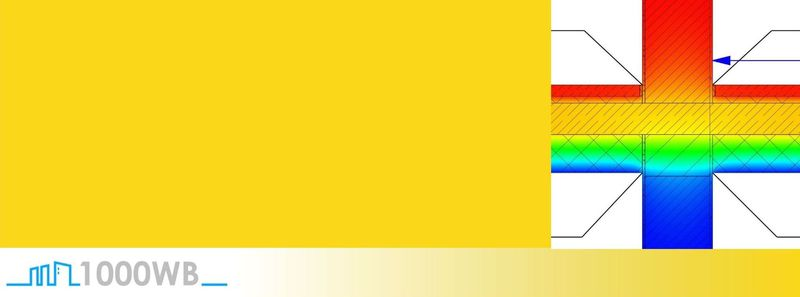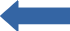# Reference lengths of the U-values – example joint outer wall – inner wall:

Posted on: May 31, 2020 Posted by: Jörg Gerl Comments: 0Here we have a thermal bridge that has a precisely defined thermal bridge coefficient for a given geometry Ψ.

This applies to the undisturbed area (the area which does not show any influence of adjacent components on the heat flow):
– U1: U-value, which is used in the heat requirement calculation as the U-value of the thicker outer wall (in this example: 0.1331 W/m²K)
– U2: U-value that is used in the heat requirement calculation as the U-value of the thinner outer wall (in this example: 0.1392 W/m²K)

This geometry assumes that in the heat requirement calculation the undisturbed U-value, which is set for the thinner wall, extends to the end of this wall.

The dimensioning and reference lengths of the U-values correspond. It is not necessary to adjust the reference lengths of the U values.

Here the U-value of the thinner wall up to and including the inner wall is calculated for the heat requirement calculation.

Dimensioning and reference lengths of the U-values no longer match. They differ by the thickness of the inner wall (11.5 cm). This results in an incorrect result in the heat requirement calculation.

The reference lengths of the U-values must be adjusted. The calculated energy requirement of the entire building changes. If the reference lengths of the U-values used in the thermal bridge calculation were not adjusted, an error would result.

By adjusting the reference lengths of the U-values (here +11.5 cm for U1 and -11.5 cm for U2) an incorrect result of the heat requirement calculation is avoided. Dimensioning and reference length of the U-values again match.

Let’s look at the matter arithmetically by inserting the values into the formula

ψ = L2D – (Σ Ui*lI*Fxi)

or here specifically:

ψ = L2D – (U1*l1*Fx1+ U2*l2*Fx2)

Case one:
In this example we have the following sizes:

L2D = 0.7669
U1 = 0.177 W/m²K
l1 = 1.715 m
Fx1 = 1
U2 = 0.2825 W/m²K
l2 = 1,600 m
Fx2 = 1

This results in Ψ = 0.0114 W/mK

Case two:
If we now change the reference lengths of the U-values by the thickness of the inner wall (11.5 cm), it looks like this:

L2D = 0.7669
U1 = 0.177 W/m²K
l1 = 1.715 m – 0.115 m = 1.60 m
Fx1 = 1U2 = 0.2825 W/m²K
l2 = 1.600 m + 0.115 m = 1.715 m
Fx2 = 1

This results in Ψ = -0.0008 W/mK

This value is better than the unchanged Ψ value and thus takes into account the fact that a larger wall area with a poorer U-value causes more energy loss compared to the original case.